Published: 15 February 2018

# Bifurcation and chaotic behaviors of 4-UPS-RPS high-speed parallel mechanism

Xiulong Chen1
Yonghao Jia2
1, 2College of Mechanical and Electronic Engineering, Shandong University of Science and Technology, Qingdao, 266590, China
Corresponding Author:
Xiulong Chen
Views 64

#### Abstract

In order to grasp the nonlinear characteristics of high-speed spatial parallel mechanism, the bifurcation and chaotic behaviors of 4-UPS-RPS mechanism are analyzed. Firstly, the nonlinear elastic dynamic model of the mechanism is established by using the Lagrange equation and the finite element method. Then the effects of parameters including driving angular velocity, the radius of motion trajectory, the material of driving limbs, the diameter of driving limbs, and the mass of moving platform, on the bifurcation and chaotic behaviors of high-speed spatial parallel mechanism are studied. The results show that the above parameters all have a certain influence on nonlinear characteristics of the 4-UPS-RPS high-speed spatial parallel mechanism. The research can provide important theoretical basis for the further research on the non-linear dynamics of spatial parallel mechanism.

## 1. Introduction

With the development of modern industry, the requirement of the precision and dynamic performance of mechanical products is gradually increased. Nowadays, parallel mechanism has received more and more attention [1, 2]. Compared with the serial mechanism, parallel mechanism has the characteristics of high speed, high precision and no cumulative error. Chen et al.  analyzed the kinematics of 4-UPS-RPS spatial 5-DOF parallel mechanism. Liu et al.  studied the kinematics and dynamics of a spatial 3 degree-of-freedom parallel manipulator. Sun et al.  established a dynamic model of high-speed 6-PSS parallel robot and optimized the parameters of the mechanism.

There are a wide range of nonlinear factors in the mechanisms, such as clearance, dry friction, bearing oil film and nonlinear material constitutive relation [6-10]. As a seemingly irregular and random phenomenon, chaos is one of the most important nonlinear characteristic caused by nonlinear factors [11-14]. Recently, the chaotic behavior analysis of the mechanism is mainly focused on the time-delay system, vibro impact system, the mechanism with joint clearances and so on. G. I. Koumene Taffo et al.  studied the effects of time delay on the stability of the nonlinear oscillator and analyzed the heteroclinic bifurcation of the system by the Melnikov method. Wu et al.  analyzed the bifurcation and chaotic anti-control of a 3 degree-of-freedom vibro-impact system with clearance. Lu et al.  studied the influence of clearance to the steering swing system, and concluded that the pair clearance can make the swing system from the state of periodic motion, quasi periodic motion to chaotic motion gradually. As is known to all, nonlinear deformation of light components of high-speed parallel mechanism can cause complex chaotic behaviors, and worsen the dynamic performance of the mechanism. But up to now, the previous studies were focused on the nonlinearity caused by clearance, dry friction and bearing oil film, rarely involved the bifurcation and chaotic behaviors analysis of spatial high-speed parallel mechanism with nonlinear deformation of the limbs.

Therefore, the main purpose of this paper is to study the bifurcation and chaotic behaviors of spatial high-speed parallel mechanism, thus forecasting the dynamic behavior of the parallel mechanism and selecting appropriate system parameters of the mechanism. This paper regards 4-UPS-RPS high-speed spatial parallel mechanism as the research object. The nonlinear elastic dynamic model of the mechanism is introduced. The bifurcation and chaotic behaviors of the 4-UPS-RPS high-speed parallel mechanism with different parameters are analyzed by the phase diagram, the Poincare map, bifurcation diagram and the largest Lyapunov exponent method.

## 2. Description of high-speed spatial parallel mechanism

4-UPS-RPS (U represents hooke hinge, P represents prismatic pair, S represents spherical joint, R represents revolute pair) high-speed spatial parallel mechanism is shown in Fig. 1. The mechanism is composed of a fixed platform, a movable platform and five driving limbs. The fixed platform is connected with the movable platform by four UPS branch and a RPS. By changing the five driving limbs length, the movable platform can realize three-dimensional rotation and the two dimensional translation. The coordinate system of 4-UPS-RPS high-speed spatial parallel mechanism is shown in Fig. 2.

Fig. 1Mechanism diagram of 4-UPS-RPS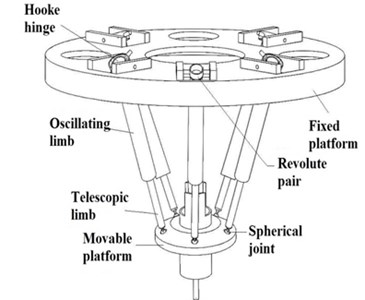Fig. 2The coordinate system of 4-UPS-RPS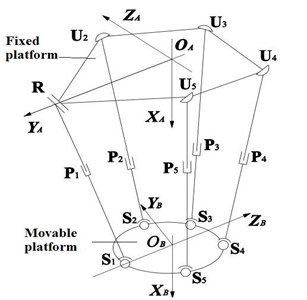## 3. Nonlinear elastic dynamic model of high-speed spatial parallel mechanism

According to the Lagrange equation and the finite element method, the nonlinear elastic dynamic model of the spatial high-speed parallel mechanism is given by :

1
$M\stackrel{¨}{q}+\stackrel{~}{C}\stackrel{˙}{q}+Kq=Q,$

where $M={\sum }_{i=1}^{5}{R}_{{i}_{}}^{T}{M}_{i}{R}_{i}+{R}_{0}^{T}{M}_{0}{R}_{0}$ is the system total mass matrix, $\stackrel{~}{C}={\sum }_{i=1}^{5}{R}_{i}^{T}{C}_{i}{R}_{i}$ is the system total damping matrix, $K={\sum }_{i=1}^{5}{R}_{i}^{T}{K}_{i}{R}_{i}$ is the system total stiffness matrix, $Q={\sum }_{i=1}^{5}{R}_{i}^{T}{Q}_{i}+{R}_{0}^{T}{Q}_{0}$ is the system total generalized force matrix, $q$ is the generalized coordinate of system, ${R}_{i}$ is the transfer matrix from system to driving limbs, ${R}_{0}$ is the transfer matrix from system to moving platform.

## 4. Bifurcation and chaotic behaviors analysis of high-speed spatial parallel mechanism

As we all known, we can judge whether the system is chaotic or not by observing the intercept point on the Poincare map [19, 20]. When the Poincare map is only one fixed point or a small number of discrete points, the system is in periodic motion. When the Poincare map is the limit cycle, the system is in almost periodic motion. When the Poincare map is stretches of dense point and having a fractal structure, the system is in chaotic motion .

Chaotic motion is very sensitive to the initial condition. Lyapunov exponents are a quantitative description of the indicators of this phenomenon . When the Lyapunov exponent is negative in the direction, the phase volume is shrinkage, and the motion is stable. When the Lyapunov exponent is positive in the direction, the motion is in chaotic state. If the largest Lyapunov exponent (LLE) is positive, the system must be chaos. Therefore, the largest Lyapunov exponent of the time series can be used as a criterion for determining whether a sequence in a state of chaos [23, 24].

There are many methods for solving the largest Lyapunov exponent, such as the definition, the small data method, the Wolf method and the Jacobian method. The Wolf method is widely used in the research of chaos behaviors and chaotic time series prediction based on Lyapunov exponent [25-28]. The nonlinear elastic dynamic model shown in Eq. (1) is a set of coupled variable coefficient second order differential equations, and as everyone knows, this kind of dynamic model can be solved by Newmark integral method, and the Lyapunov exponent can be estimated by Wolf method . Then in this paper, the Lyapunov exponent is estimated by Wolf method. The main calculation steps of Wolf method are as follows:

Step 1. Estimated average period by using fast Fourier transform.

Step 2. Estimated time delay and embedding dimension by using C-C method.

Step 3. Reconstructed phase space by using the time delay technique.

Step 4. Obtained the largest Lyapunov exponent by mapping the Lyapunov exponent chart and looked for stable region data.

The distribution of hinge of 4-UPS-RPS parallel mechanism is shown in Table 1. The basic parameters of 4-UPS-RPS parallel mechanism are shown in Table 2. The law of motion for 4-UPS-RPS spatial parallel mechanism is defined as (unit: rad, m):

2
$\left\{\begin{array}{l}X=1.280+r×\mathrm{c}\mathrm{o}\mathrm{s}\left(\omega t\right),\\ Y=0.260+r×\mathrm{s}\mathrm{i}\mathrm{n}\left(\omega t\right),0\le t\le 600\mathrm{}\text{s},\\ \alpha =0,\\ \beta =0,\\ \gamma =0.\end{array}\right\$

Table 1The distribution of hinge of 4-UPS-RPS parallel mechanism (mm)

 Hinge type R U2 U3 U4 U5 S1 S2 S3 S4 S5 Distribution radius 717.07 645 645 645 645 202 202 202 202 202 Distribution angle 0 $\pi /4$ $3\pi /4$ $5\pi /4$ $7\pi /4$ 0 $2\pi /5$ $4\pi /5$ $6\pi /5$ $8\pi /5$

Table 2The basic parameters of 4-UPS-RPS parallel mechanism

 The length of oscillating limb 760 mm Elastic modulus of tension and compression $E=$2.0×1011 Pa The length of telescopic limb 840.116 mm Shear elastic modulus $G=$8.0×1010 Pa The cross section radius of telescopic limb 20 mm Poisson ratio 0.29 The material of telescopic limb Steel The density of telescopic limb 7.801×103 kg/m3 The material of moving platform Aluminum alloy The mass of moving platform ${m}_{0}=$ 36.28 kg Principal moment ofinertia of unit crosssection to $x$-axis,$y$-axis and $z$-axis,respectively ${I}_{x}=$ 2.512×10-7 m4 Moment of inertia of moving platform ${I}_{xx}=$ 0.932 kg·m2 ${I}_{xy}=$9.549×10-3 kg·m2 ${I}_{xz}=$ –1.937×10-2 kg·m2 ${I}_{yx}=$9.549×10-3 kg·m2 ${I}_{y}=$ 1.256×10-7 m4 ${I}_{yy}=$0.6735 kg·m2 ${I}_{yz}=$8.43×10-4 kg·m2 ${I}_{z}=$1.256×10-7 m4 ${I}_{zx}=$–1.937×10-2 kg·m2 ${I}_{zy}=$8.43×10-4 kg·m2 ${I}_{zz}=$0.6816 kg·m2

### 4.1. Effect of driving angular velocity on the dynamic behavior of high-speed spatial parallel mechanism

When the other parameters are invariant and $r=$0.045, we change the values of the driving angular velocity, the phase diagram of the system in $X$ direction and the corresponding Poincare map are shown from Fig. 3 to Fig. 8. Bifurcation diagram of displacement with driving angular velocity is shown in Fig. 9.

Fig. 3The phase diagram and Poincare map when ω=π rad/s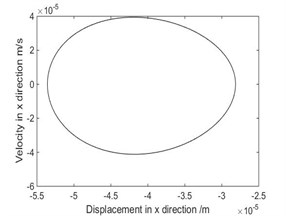a) Phase diagram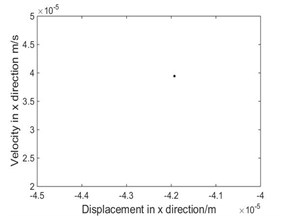b) Poincare map

Fig. 4The phase diagram and Poincare map when ω= 4.712 rad/s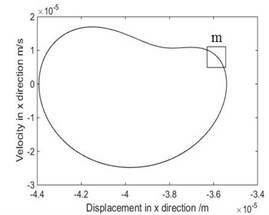a) Phase diagram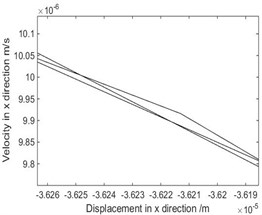b) Local phase diagram of $m$ region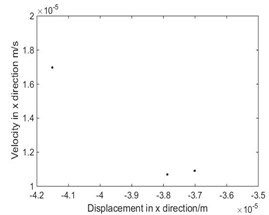c) Poincare map

Fig. 5The phase diagram and Poincare map when ω= 9.2 rad/s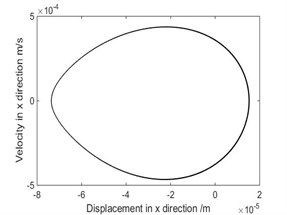a) Phase diagram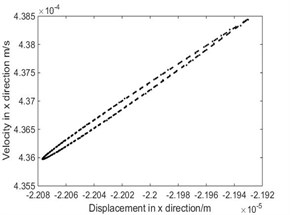b) Poincare map

As shown in Fig. 3, when $\omega =\pi$ rad/s, phase diagram has only one trajectory and Poincare map has only one point, the system is in single-cycle motion. When $\omega$ is 4.712 rad/s, the system becomes unstable and bifurcates from single-cycle to 3-cycle. From Fig.4, when $\omega =\mathrm{}$4.712 rad/s, phase diagram has three tracks and Poincare map has three points, the system is in a state of three periodic motion. As shown in Fig. 5, when $\omega$ is 9.2 rad/s, phase diagram has many trajectories and Poincare map is a limit cycle, the system is in a state of almost periodic motion. From Fig. 6, when $\omega =$ 9.35 rad/s, phase diagram has five tracks and Poincare map has five points, the system appears 5-cycle. As shown in Fig. 7, when $\omega =$ 9.4 rad/s, the system is in a state of almost periodic motion. From Fig. 8, when $\omega =$ 9.417 rad/s, the almost periodic ring is fracture, and the system is transferred from almost periodic motion to chaos, the phase trajectory of the system is not repeated and the Poincare map is a bit of dense points with fractal structure. The results show that the driving angular velocity has a great influence on the motion state of the system. When driving angular velocity is different, the system has different motion state. Overall, from Fig. 9, we could see the effects of driving angular velocity on chaotic behavior of the parallel mechanism.

Fig. 6The phase diagram and Poincare map when ω= 9.35 rad/s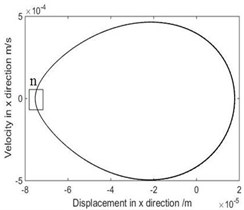a) Phase diagram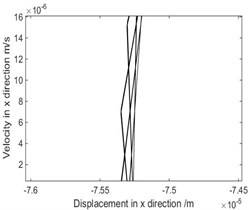b) Local phase diagram of $m$ region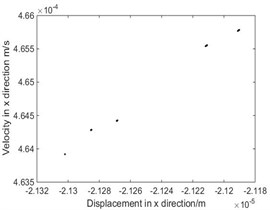c) Poincare map

Fig. 7The phase diagram and Poincare map when ω= 9.4 rad/s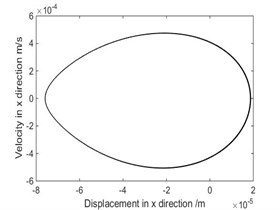a) Phase diagram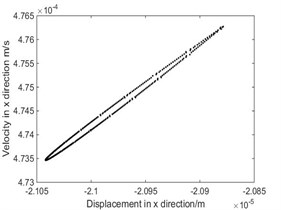b) Poincare map

Fig. 8The phase diagram and Poincare map when ω= 9.417 rad/s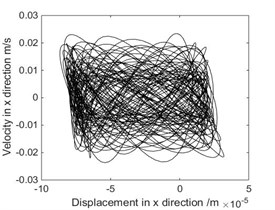a) Phase diagram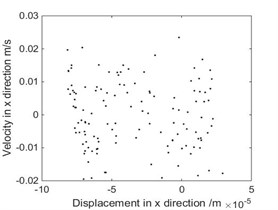b) Poincare map

Fig. 9Bifurcation diagram of displacement with driving angular velocity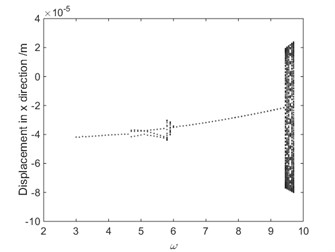Fig. 10Bifurcation diagrams of largest Lyapunov exponent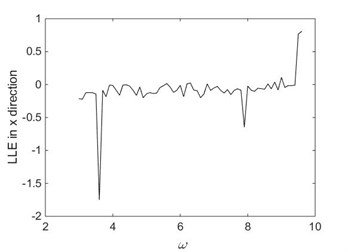Fig. 11Lyapunov exponent chart in X direction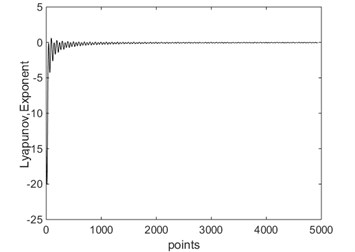a) LLE = –0.2145 when $\omega =\pi$ rad/s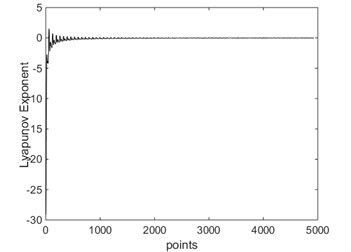b) LLE = –0.1593 when $\omega =$ 4.712 rad/s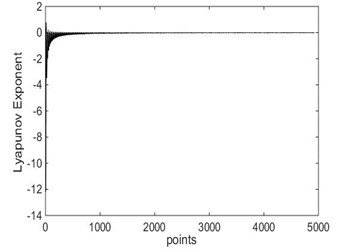c) LLE = –0.0155 when $\omega =\mathrm{}$9.2 rad/s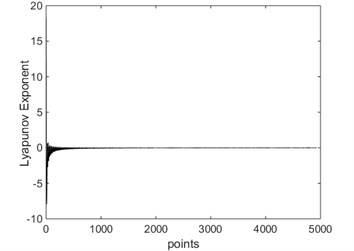d) LLE = –0.0455 when $\omega =\mathrm{}$9.35 rad/s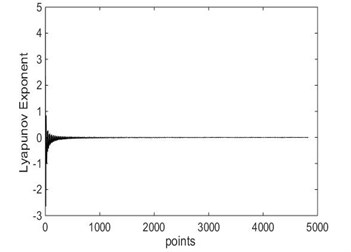e) LLE = –0.0099 when $\omega =\mathrm{}$9.4 rad/s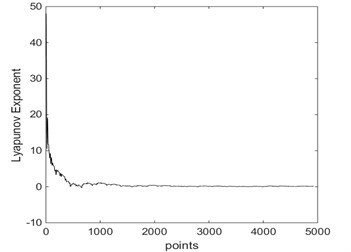f) LLE = 0.7749 when $\omega =\mathrm{}$9.417 rad/s

Bifurcation diagrams of largest Lyapunov exponent is shown in Fig. 10. From Fig. 10, when $\omega <$ 9.417 rad/s, the largest Lyapunov exponent in X direction is negative; when $\omega \ge$ 9.417 rad/s, the largest Lyapunov exponent in $X$ direction is positive. The Lyapunov exponent diagram in $X$ direction corresponding to different driving angular velocity is shown in Fig. 11. From Fig. 11, when $\omega =\pi$ rad/s, $\omega =$ 4.712 rad/s and $\omega =\mathrm{}$9.35 rad/s, the largest Lyapunov exponent in $X$ direction are –0.2145, –0.1593 and –0.0455, respectively. In these cases, the system is in a state of periodic motion. When $\omega =$ 9.2 rad/s and $\omega =$ 9.4 rad/s, the largest Lyapunov exponent in $X$ direction are –0.0155 and –0.0099, respectively. And the system is in a state of almost periodic motion. When $\omega =$ 9.417 rad/s, the largest Lyapunov exponent in $X$ direction is 0.7749, the Lyapunov exponent is positive, therefore the system is in a state of chaos. As shown in Fig. 10 and Fig. 11, the driving angular velocity has a complicated influence on the chaotic behaviors of the 4-UPS-RPS high-speed parallel mechanism.

### 4.2. Effect of the radius of motion trajectory on the dynamic behavior of high-speed spatial parallel mechanism

Due to the limitation of the workspace of the 4-UPS-RPS mechanism, the radius of motion trajectory denoted as $r$ is choosed from 0.038 m to 0.057m. When $\omega =\pi$ rad/s, bifurcation diagram of displacement with the radius of motion trajectory is shown in Fig. 12. From Fig. 12, the system is stable.

Fig. 12Bifurcation diagram of displacement with the radius of motion trajectory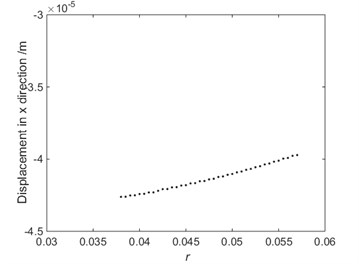### 4.3. Effect of the material of driving limbs on the chaotic motions of parallel mechanism

When $\omega =$ 9.417 rad/s and $r=$ 0.045, the largest Lyapunov exponent in $X$ direction of two different materials is shown in Fig. 13. If the driving limbs were aluminum, the largest Lyapunov exponent in $X$ direction is –0.2808. If the driving limbs were copper, the largest Lyapunov exponent in $X$ direction is –0. 1092. From Fig. 13, the system is stable.

Fig. 13Lyapunov exponent in X direction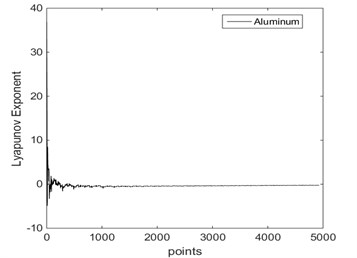a) Aluminum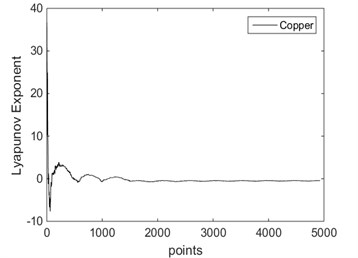b) Copper

### 4.4. Effect of diameter of driving limbs and the mass of moving platform on the bifurcation and chaotic motions of parallel mechanism

When $\omega =$ 9.417 rad/s, $r=$0.045 and the materials of the driving limbs was steel, the relationship between the LLE in $X$ direction and the diameter of driving limbs is shown in Fig. 14, the corresponding bifurcation diagram of displacement with the diameter of driving limbs is shown in Fig. 15. From Fig. 15, the system is in chaotic state. When $\omega =$ 9.417 rad/s, $r=$ 0.045 and the materials of the driving limbs was steel, the relationship between the LLE in $X$ direction and the mass of moving platform is shown in Fig. 16. The corresponding bifurcation diagram of displacement with the mass of moving platform is shown in Fig. 17. As shown in Fig. 17, the system is sometimes in periodic state and sometimes in chaotic state. From Fig. 14 to Fig. 17, the diameter of driving limbs and the mass of moving platform have a complex influence on the chaotic motions of the 4-UPS-RPS high-speed parallel mechanism.

Fig. 14The relationship between the diameter of driving limbs and the LLE in X direction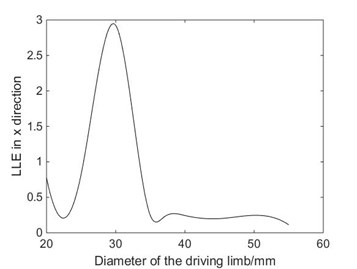Fig. 15Bifurcation diagram of displacement with the diameter of driving limbs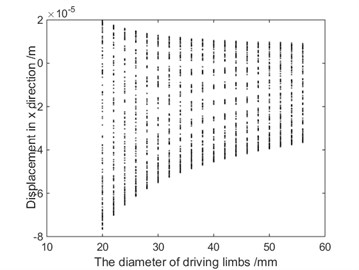Fig. 16The relationship between the mass of platform and the LLE in X direction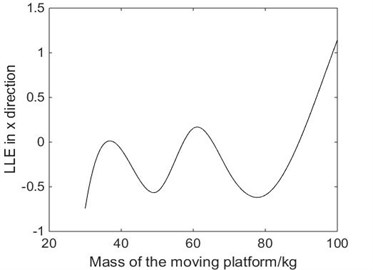Fig. 17Bifurcation diagram of displacement with the mass of moving platform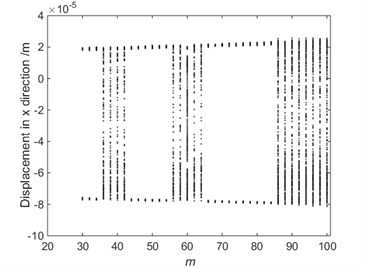## 5. Conclusions

This paper investigates the bifurcation and chaotic behaviors of 4-UPS-RPS high-speed spatial parallel mechanisms. The main conclusions are as follows:

1) The nonlinear elastic dynamics model of 4-UPS-RPS high-speed spatial parallel mechanism is introduced.

2) The effects of parameters including driving angular velocity, the radius of motion trajectory, the material of driving limbs, the diameter of driving limbs, and the mass of moving platform, on the bifurcation and chaotic behaviors of high-speed spatial parallel mechanism are studied by phase diagrams, Poincare map, bifurcation diagram and largest Lyapunov exponent, respectively. The results show that the above parameters all have a certain influence on the nonlinear characteristics of the 4-UPS-RPS high-speed spatial parallel mechanism.

3) The results show that the chaos phenomena can be avoided by selecting suitable control parameter, and therefore the motion property of mechanism is improved. The research can provide important theoretical basis for the further research on the nonlinear characteristic of 4-UPS-RPS high-speed spatial parallel mechanism.

20 August 2017
Accepted
21 December 2017
Published
15 February 2018
SUBJECTS
Chaos, nonlinear dynamics and applications
Keywords
high-speed
parallel mechanism
bifurcation
chaotic behaviors
Acknowledgements

This research is supported by the Natural Science Foundation of Shandong Province (Grant No. ZR2017MEE066), the Shandong Young Scientists Award Fund (Grant No. BS2012ZZ008).